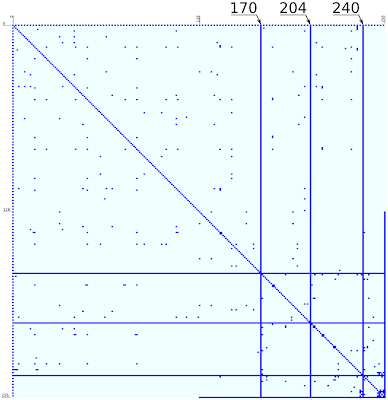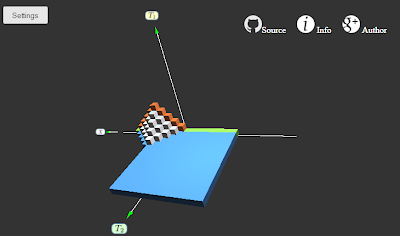# Two dimensions of time in cellular automataEvoution of the cellular automata with 2 temporal dimensions. Initial pattern is single cell (green). Rule 150 acts along time $$T_1$$, and Rule 60 - along $$T_2$$. See the Simulator section below or try it now.

Our world have 3 (observable) spatial dimensions and 1 time dimension. It is easy for us to reason about a world with 1 or 2 spatial dimensions, and with some effort, imagining a world with 4 spatial dimensions is not impossible too:

Larger dimensions: 5 and more are easy to describe mathematically, though my geometrical intuition raises hands here.

Now, what about time dimensions? We often silently assume that time is one-dimensional: each moment of time is represented by a single real number. Can we reason about several time dimensions?

Seems that for physicists, this idea is not something new: What would the universe be like with additional temporal dimensions?. But I will use a simpler approach and try to explore it using cellular automata as a simplified model of physics.

Cellular automata are good examples of “toy physics”, that we know bottom-up. In the real word, we observe complex phenomena and can only deduce underlying principles (that's what physicists do). In the world of cellular automata the situation is the opposite: basic rules are given but the behavior of complex systems is a subject of research.

How to imagine a cellular automaton with several dimensions of time? Here are some of my ideas and code.

## Time in cellular automata

Ordinary cellular automaton is defined by the world state and transition function (rule). World state is a combination of the states of all cells in the grid, and transition function defines, how the state changes.Time in the regular cellular automata: sequence of world states, connected by the transition function.

The evolution of the world state is determined by the initial state $$S_0$$ and transition function $$S_{t+1} = f(S_t)$$. Naturally, in this simple deterministic world, a moment of time can be thought as a nonnegative integer $$t \in \mathbb{Z}^{*}$$ which is the number of applications of the transition function. (It can't be negative because $$f(S)$$ is not always invertible). The time can also be seen as a path from the original state $$S_0$$ to some other state $$S$$. Since we can only move in time in one direction, this path is, literally, straightforward. The “structure” of the time set is simple: each moment have one successor.

## Two-dimensional time

The above definition is rather trivial, but it gives a hint how to extend the notion of time to several dimensions.

Let the time value be 2-dimensional, a pair of nonnegative integers: $$t=(t_1,t_2) \in \mathbb{Z}^{*2}$$. Now each moment $$(t,\tau)$$ have 2 direct successors: $$(t+1,\tau)$$, $$(t,\tau+1)$$. Thus, there should be two transfer functions: $$S_{t+1,\tau} = f(S_{t,\tau}),$$ $$S_{t,\tau+1} = g(S_{t,\tau}).$$

Way too easy, isn't it? Putting aside all the nicely rendered formulas and blah-blah-blah, the idea is just this simple: in order to have 2 arrows of time, one must have 2 ways to calculate future states.

Well, it appears that there is one more important complication: rules must be consistent with each other. Look at the figure below:

The same future state $$S_{2,2}$$ can be reached from the initial state $$S_{0,0}$$ in the $$\binom 4 2 = 6$$ different ways. If the notion of 2-time is well-defined, all these time paths must always give the same result, for every initial state $$S_{0,0}$$: $$f(f(g(g(S_{0,0})))) =$$ $$= f(g(f(g(S_{0,0})))) = ...$$ It is true iff the transfer functions $$f$$ and $$g$$ commute :

As will be shown later, this consistency condition poses significant limitations on the transfer functions.

## Consistent automata with 2 time and 1 space dimensions

So, I tried to find to find a pair of commuting cellular automata to build an automaton with 2 time dimensions. To simplify the task, I've limited my search to the elementary CA, 1-dimensional automata with 2 states and neighborhood of 2 cells. Since there are only $$2^8=256$$ of them, commuting pairs can be found trivially, by complete enumeration. To check whether 2 automata commute, it is enough to check that they commute on every initial pattern of 5 cells.

And, here is what I have found: the map of all pairs of commuting elementary cellular automata. Click to open it wide; here are the raw data for this table, one line for each pair of commuting CAs, excluding identical rules.Map of the commuting elementary cellular automata pairs. Coordinate is the automata index, commuting pairs are shown in blue. (Clickable). See this post to see this map rendered as graph!

The above map displays several distinguishable features.

### Trivial cases

#### Rule 170, rule 204, rule 240.

These 3 rules commute with every other rule. This is easy to explain, because Rule 204 is an identity rule, leaving the world unchanged; and rules 204, 240 are “translation” rules shifting the world by 1 cell to the left and to the right.

#### Rule 0

The first row and column. Rule 0 turns all cells to 0 regardless of its previous state and neighbors. Map shows that is commutes with even rules: rules that preserve emptiness of the space.

#### Rule 255

This is an opposite of the rule 0. Rule 255 fills world with ones regardless of the previous state. It commutes with rule numbers 128...255, that are rules, preserving world filled with 1s.

#### The diagonal

The diagonal illustrates a trivial fact: every rule commutes with itself.

Alas, all of the above-mentioned rule pairs are not very interesting. Their commutativity is a trivial fact and they will not produce any interesting CA with 2-dimensinal time

Additive automata have rules, compatible with “addition” of their states, where “addition” can refer to any associative binary operation: AND, OR, XOR. (See Wolfram article on them) $$f(S_1+S_2) = f(S_1) + f(S_2).$$ Any two automata that are additive regarding to the same operation, always commute. For example, Rule 90 which is additive under the OR operation, commutes with rules 60, 102 and 150, which are all additive under the OR.

### Other cases

There are commuting rule pairs besides the trivial cases and additive rules. Some examples are:

• Rule 50 and Rule 178.
• Rule 4 and Rule 42
• Rule 32 and Rule 128 (the latter is additive under AND, but the former is not).
• and many others.

## Conclusion: slightly disappointing

At the first glance, it seems that plenty of commuting CA pairs exist, the raw table contains 1164 non-trivial pairs. Unfortunately, the most interesting chaotic automata:

• chaotic Rule 30, and
• Rule 110, which can be used to build Turing machine,
don't commute with any other rule (excluding the trivial cases). Most commuting pairs are additive in relation to some boolean operation, and non-additive example still have very simple Class-1 behavior, like the 32-232 pair.

This means that nontrivial elementary automata with 2 temporal dimensions do not support complex behavior. Which is indeed a sad discovery, at least for me.

# Online Simulator

You can explore the behavior of cellular automata with 2d time in the online simulator. It runs in the browser and is based on the Three.js engine, using WebGL technology.

## Interface

On the main screen a 3-d representation of the evolution of the initial 1-d pattern along 2 different time axes. Initial pattern is shown with green boxes, by default it is a single box. Red boxes represent evolution purely along axis $$T_1$$, and blue boxes - $$T_2$$.Main screen of the simulator. Rule pair is 32-232, initial pattern: "#_#_#_#_#_#########".

To select rule pair and initial pattern, click the [Settings] button that opens settings screen. Here, you can select 2 elementary cellular automata rules. Rule along the $$T_1$$ axis can be chosen freely, and rule along $$T_2$$ is constrained to be compatible with the first one. Rules can be chosen either by their number, or using rule map:Rules map, showing 128 rules with even indices. Rule indices increase from the top to the bottom, and from the left to the right.

On this map:

• Cell background color indicates number of compatible rules. The lighter it is, the more compatible rules are present.
• Green border around the cell indicates rule, described at the Wolfram site. When you select it, a link appears.
• Yellow icons indicate rule additivity (several icons can be present).
• [∨] : additive relative to logical OR.
• [∧] : additive relative to logical AND.
• [+] : additive relative to logical XOR.

Near the map, there is a diagram, showing evolution of the given initial pattern according to the selected rule. Initial pattern could be entered below, using "#" for the “alive” cells and any other character for the “dead” ones.

“Simulation time” field determines for how long (in each time direction) simulation should be done. It determines size of the generated 3d object. Be careful, the simulator is not highly optimized, and high value could slow it down significantly.

## Sources

Sources of the simulator and some Python tools are hosted at Github under permissive license. Code is written in Coffeescript, and with Tree.js framework used for 3d graphics. There are also some tools, written in Python.